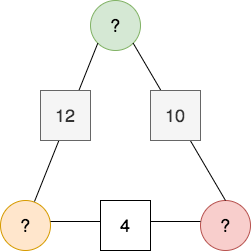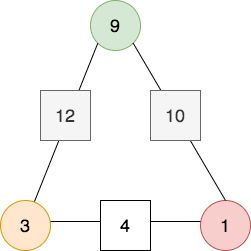# Arithmagons

## What is arithmagon?

An arithmagon is a polygon with numbers at its vertices which determine the numbers written on its edges.

Total in the square is a sum of two circles where square is attached.Find out values in the circle.

## Solving a problem

Let's say top vertex is x and moving in clockwise bottom right vertex is y and then bottom left is z as shown in the figure below:Let's assume:

Let's take two equation to solve the problem

Now, we can say following:

if

Adding x value in equation so that

Now, that we have value of z we can find all values

Now, we have y=1 solve the following equation and get x

Now, we have x=9 solve the following equation and get x

Let's place our values in circles and test the answer:This math problem can be solved in different ways to checkout other ways check the following link:

Reference: Nrich.Maths.Org